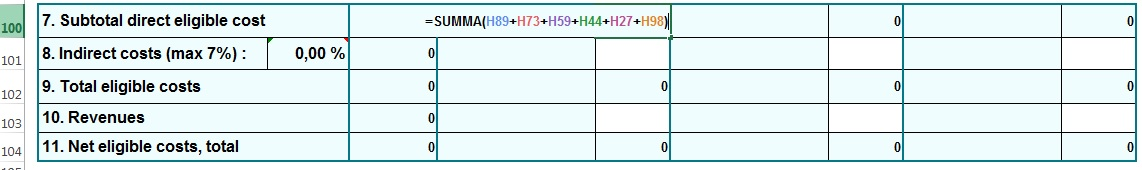# Template of Annex A: Budget Specification update 14.2.2017

Published: February 16, 2017

Template of Annex A: Budget Specification were updated on 14.2.2017. Please download new template Applying – Guidelines for applicants – Documents for applying

Annex A Budget specification_update 14.2.2017

• Corrected formula in the sheets “1.1. Budget Finland”, “1.2. Budget Russia”, “1.1. Budget Sweden”, “1.1. Budget Norway” in cells H100, L100, P100. The “7. Subtotal direct eligible costs” were calculated wrong  (sum excluded 2.Travel costs).

How to correct formula by yourself

If you already have filled in the Annexes A: Budget Specification you can make the correction of formula yourself:

1. Open your file, go to sheet “1.1. Budget Finland”

2. Unprotect Sheet (on the Review tab, in the Changes group, click Unprotect Sheet)

3. Select sell:

• H100

The formula was =SUMMA(H89+H73+H59+E44+H27+H98), put new formula =SUMMA(H89+H73+H59+H44+H27+H98)

• L100

The formula was =SUMMA(L89+L73+L59+I44+L27+L98), put new formula =SUMMA(L89+L73+L59+L44+L27+L98)

• P100

The formula was =SUMMA(P89+P73+P59+M44+P27+P98), put new formula =SUMMA(P89+P73+P59+P44+P27+P98)4. Correct formulas in the sells H100, L100, P100 also in the sheets “1.2. Budget Russia”, “1.1. Budget Sweden”, “1.1. Budget Norway” as described above.

5. After the changes are done please remember to Protect Sheet again – Review tab, in the Changes group, click Protect Sheet

6. Save file